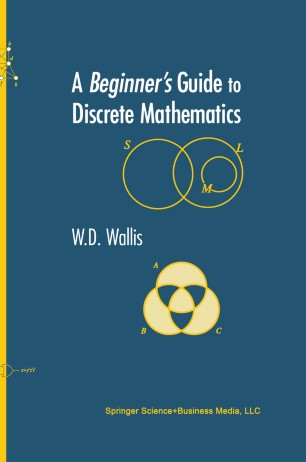# A Beginner’s Guide to Discrete MathematicsTextbook

1. Front Matter
Pages i-xiii
2. W. D. Wallis
Pages 1-30
3. W. D. Wallis
Pages 31-64
4. W. D. Wallis
Pages 65-89
5. W. D. Wallis
Pages 91-109
6. W. D. Wallis
Pages 111-154
7. W. D. Wallis
Pages 155-204
8. W. D. Wallis
Pages 205-249
9. W. D. Wallis
Pages 251-284
10. W. D. Wallis
Pages 285-326
11. Back Matter
Pages 327-367

### Introduction

This introduction to discrete mathematics is aimed primarily at undergraduates in mathematics and computer science at the freshmen and sophomore levels. The text has a distinctly applied orientation and begins with a survey of number systems and elementary set theory. Included are discussions of scientific notation and the representation of numbers in computers. An introduction to set theory includes mathematical induction, and leads into a discussion of Boolean algebras and circuits.

Relations and functions are defined. An introduction to counting, including the Binomial Theorem, is used in studying the basics of probability theory. Graph study is discussed, including Euler and Hamilton cycles and trees. This is a vehicle for some easy proofs, as well as serving as another example of a data structure. Matrices and vectors are then defined. The book concludes with an introduction to cryptography, including the RSA cryptosystem, together with the necessary elementary number theory, such as the Euclidean algorithm.

Good examples occur throughout, and most worked examples are followed by easy practice problems for which full solutions are provided. At the end of every section there is a problem set, with solutions to odd-numbered exercises. There is a full index.
A math course at the college level is the required background for this text; college algebra would be the most helpful. However, students with greater mathematical preparation will benefit from some of the more challenging sections.

### Keywords

Combinatorics DES Discrete Mathematics Graph Graph theory Probability and Statistics Probability theory Set Theory algorithms cryptography ksa

#### Authors and affiliations

1. 1.Department of MathematicsSouthern Illinois UniversityCarbondaleUSA

### Bibliographic information

• Book Title A Beginner’s Guide to Discrete Mathematics
• Authors W.D. Wallis
• DOI https://doi.org/10.1007/978-1-4757-3826-1
• Copyright Information Birkhäuser Boston 2003
• Publisher Name Birkhäuser, Boston, MA
• eBook Packages
• Softcover ISBN 978-0-8176-4269-3
• eBook ISBN 978-1-4757-3826-1
• Edition Number 1
• Number of Pages XIII, 367
• Number of Illustrations 9 b/w illustrations, 0 illustrations in colour
• Topics
• Buy this book on publisher's site

## Reviews

"Wallis's book on discrete mathematics is a resource for an introductory course in a subject fundamental to both mathematics and computer science, a course that is expected not only to cover certain specific topics but also to introduce students to important modes of thought specific to each discipline . . . Lower-division undergraduates through graduate students.

—CHOICE

"Very appropriately entitled as a 'beginner's guide', this textbook presents itself as the first exposure to discrete mathematics and rigorous proof for the mathematics or computer science student."

—ZENTRALBLATT MATH

"This book introduces the basic topics of discrete mathematics to students of mathematics and computer science. … It is appropriate for first-year students in mathematics and computer science. Sample problems and solutions are presented throughout the text. … In addition, the book provides many exercises for each section of material. … The book is very user-friendly. … If you teach discrete mathematics at the beginning level to students … I recommend that you take a look at this text."   —SIGACT News